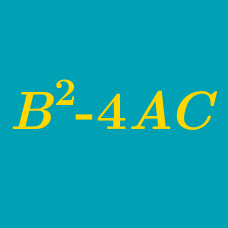Algebra

# Quadratic Discriminant: Level 3 Challenges

$f(x)=ax^2+bx+c$. $a,b,c$ are real numbers. Given that $c(a+b+c)<0$ , then what can we say about $b^2-4ac$ ?

Find the number of positive real roots of the equation $x^{4}-4x-1=0.$

Given a function $f(x) = ax^{2}-bx-16$ doesn't has 2 distinct real roots. Find the maximum value of $4a-b$.

$\prod_{k=1}^{999} (x^2-47x+k) = (x^2-47x+1)(x^2-47x+2)\dots(x^2-47x+999)$

If the product of all real roots of the polynomial above can be expressed in the form $n!$, what is the value of $n$?

Find the number of real roots of the polynomial $3x^{5} -25x^{3} +60x$.

×